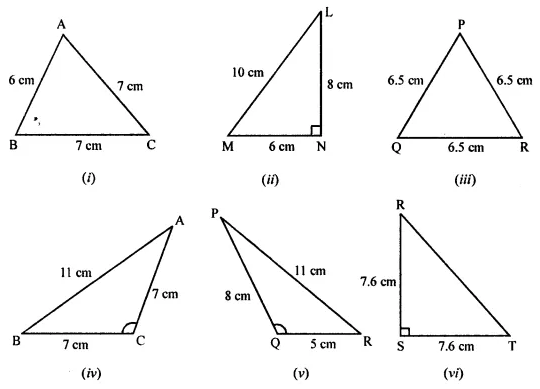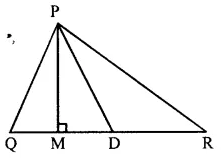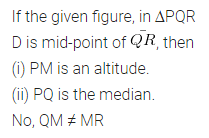# ML Aggarwal Class 7 Solutions for ICSE Maths Chapter 11 Triangles and its Properties Ex 11.1

## ML Aggarwal Class 7 Solutions for ICSE Maths Chapter 11 Triangles and its Properties Ex 11.1

Question 1.(i) Name the vertex opposite to side PQ.
(ii) Name the side opposite vertex Q.
(iii) Name the angle opposite to side QR.
(iv) Name the side opposite to ∠R.
Solution:Question 2.
Look at the figures given below and classify each of the triangles according to its (a) sides (b) angles
(You may judge the nature of the angle by observation):Solution:Question 3.
In the given ∆PQR, if D is the mid-point of $$\bar { QR }$$, then
(i) $$\bar { PM }$$ is ……..
(ii) $$\bar { PD }$$ is ………
Is QM = MR?Solution:Question 4.
Will an altitude always lie in the interior of a triangle? If no, draw a rough sketch to show such a case.
Solution:Question 5.
Can you think of a triangle in which two altitudes of the triangle is its sides? If yes, draw a rough sketch to show such a case.
Solution:Question 6.
Draw rough sketches for the following:
(i) In ∆ABC, BE is a median of the triangle.
(ii) In ∆PQR, PQ and PR are altitudes of the triangle.
(iii) In ∆XYZ, YL is an altitude in the exterior of the triangle.
Solution:Question 7.
Take an equilateral triangle and draw its medians and altitudes and check that the medians and altitudes are the same.
Solution: